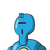# >2x = √2 + 1 / √2 – 1y = √2 – 1 / √2 + 1 find the value of x² + y²+xy = ?​

>
2x = √2 + 1 / √2 – 1
y = √2 – 1 / √2 + 1
find the value of x² + y²+xy = ?​

### 1 thought on “><br />2x = √2 + 1 / √2 – 1<br />y = √2 – 1 / √2 + 1 <br />find the value of x² + y²+xy = ?​”

1.I have got the answer is 35

Step-by-step explanation:

so we have,

x = (√2 + 1) /(√2 – 1)

or, x = (√2 + 1)(√2 + 1) / (√2 – 1)(√2 + 1)

or, x = [(√2)² + (2 × √2 × 1) + (1)²] / [(√2)² – (1)²]

or, x = [2 + 2√2 + 1] / [2 -1]

or, x = [3 + 2√2] / 1

or, x = 3 + 2√2

y = (√2 – 1) / (√2 + 1)

or, 1/y = (√2 + 1) / (√2 – 1)

or, 1/y = 3 + 2√2

or, y = 1/(3 + 2√2)

or, y = (3 – 2√2) / (3 + 2√2)(3 – 2√2)

or, y = (3 – 2√2) / [(3)² – (2√2)²]

or, y = (3 – 2√2) / [9 – 8)

or, y = 3 – 2√2

(x × y) = [(√2 + 1) / (√2 – 1)] × [(√2 – 1)(√2 + 1)] = 1

(x + y) = (3 + 2√2 + 3 – 2√2) = 6

x² + y² + xy

= (x + y)² – 2xy + xy

= (6)² – (2 × 1) + 1

= 36 – 2 + 1

= 37 – 2

= 35